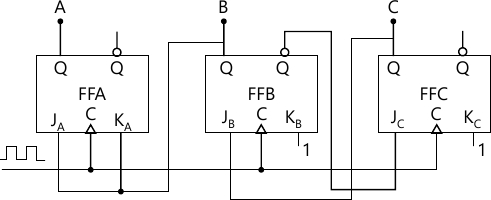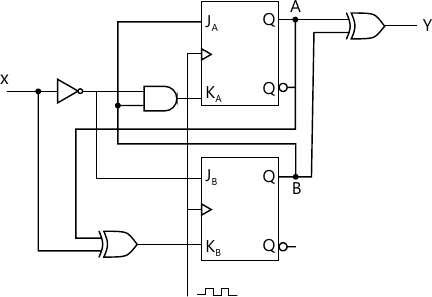Total marks: --
Total time: --
INSTRUCTIONS
(1) Assume appropriate data and state your reasons
(2) Marks are given to the right of every question
(3) Draw neat diagrams wherever necessary

1 (a) Design a three-input one-output minimal tow level gate combinational circuit which has an output equal to 1 when majority of its inputs are at logic 1 and has an output equal to 0 when majority of its inputs are at logic 0.
8 M
1 (b) Minimize the expression: $\overline{Y}= \overline{A}B\overline{C}\ \overline{D} + \overline{A} B\overline{C}D+ A B \overline{C} \ \overline{D}+AB\overline{C}D+A\overline{B}\ \overline{C}D+\overline{A} \ \overline{B}C\overline{D}$
6 M
1 (c) Reduce the following functions using K-map technique.
f{A, B, C, D}=Π M(0, 3, 4, 7, 8, 10, 12, 14) + d(2,6).
6 M

2 (a) Obtain all the prime implicants of the following Boolean function using Quine-McCluskey method.
f(a,b,c,d)=∑(0,2,3,5,8,10,11)
verify the result using K-map technique.
10 M
2 (b) Simplify the given function using MEV technique taking the least significant variable as the map entered variable.
f(a,b,c,d,e)=∑(1,3,4,6,9,11,12,14,17,19,20,22,25,27,28,30)+∑d(8,10,24,26).
10 M

3 (a) Implement the multiple functions:
f1(a,b,c,d)=∑(0,48,10,14,15) and
f2(a,b,c,d)=∑(3,7,9,13) using two 3 to 8 decoder.
6 M
3 (b) Implement the following with a suitable decoder with active to enable input and active high output: f(w,x,y,z)=∑(3,7,9)
g(a,b,c)=Π(2,4,7).
6 M
3 (c) Draw the interfacing diagram of ten key keypad interface to a digital system using decimal to BCD encoder.
6 M

4 (a) Configure a 16 to 1 MUX using 4 to 1 MUX.
6 M
4 (b) Implement the following Boolean function with 8:1 multiplexer f(A, B, C, D)=∑m(0, 2, 6, 10, 11, 12, 13)+d(3, 8, 14).
6 M
4 (c) Write a truth table for two-bit magnitude comparator. With the K-map for each output of two-bit magnitude for two-bit magnitude comparator. Write the K-Map for each output of two-bit magnitude comparator and the resulting equation.
8 M

5 (a) What do you mean by sequential circuit? Explain with the help of block diagram?
4 M
5 (b) Explain with timing diagram, the working of SR latch as a switch debouncer.
6 M
5 (c) Explain the working of a master-slave JK flip-flop with the help of logic diagram, function table, logic symbol and timing diagram.
10 M

6 (a) Obtain the characteristic equation for a SR flip-flop.
4 M
6 (b) Design a 4-bit register using positive edge triggered D flip-flops to operate as indicated in the table below:
 Mode Select Register Operation a1 a2 0 0 Hold 0 1 Synchronous clear 1 0 Complement Contents 1 1 Circular shift right
7 M
6 (c) Design a synchronous Mod-6 counter using JK flip-flop.
7 M

7 (a) Explain mealy and Moore models of a clocked synchronous sequential circuits.
8 M
7 (b) Analyse the synchronous sequential circuit shown in Fig. Q7(b).12 M

8 (a) Write the basic recommended steps for design of a clocked synchronous sequential circuit.
6 M
8 (b) Construct the excitation table, transmission table, state table and state diagram for the Moore sequential circuit shown in Fig. Q8(b).14 M

More question papers from Logic Design